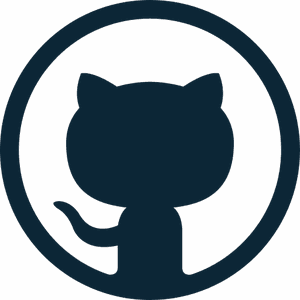# Catch up on the latest AI articles# Can You Protect Your Data From Deep Learning?

3 main points

✔️ Propose a method to create $unlearnable$ data that cannot be used by deep learning models for training
✔️ Prevent unauthorized use or misuse of personal data by deep learning models through the noise that minimizes the error
✔️ Demonstrate the effectiveness of $unlearnable$ data in various conditions

Submitted on 13 Jan 2021)
Subjects: Machine Learning (cs.LG); Cryptography and Security (cs.CR); Computer Vision and Pattern Recognition (cs.CV); Machine Learning (stat.ML)## first of all

The success of deep learning is supported by large data sets collected from the Internet. However, there is no guarantee that the data on the Internet will be collected with consent or used for legitimate purposes.

For example, selfies posted on social networking sites may be used to create a face recognition system or to create a system that identifies a person's profile based on their face photo. In this way, personal data posted on the Internet may be used without the person's knowledge and maybe misused for illegal purposes.

To address these issues, the paper in this article proposes a method for creating data that is "unlearnable" ($unlearnable$) by deep learning. This involves adding noise to the image (to the extent that it is unrecognizable to humans) so that the deep learning model that uses it for training cannot perform effectively.

It is similar to Adversarial Attack in some respects, but in this method, a characteristic feature is that the model is made to believe that there is nothing that can be learned by adding noise that minimizes the loss (error).

## technique

### Problem setting and goals

First, let's discuss the problem setup.

The assumed task is an image classification task using a deep neural network (DNN).

For the $K$-class classification task, let the clean (no $unlearnable$ data) train data be $D_c$, the test data be $D_t$, and the DNN trained on $D_c$ be $f_\theta$. (For example, the MNIST train set is denoted as $D_c$, the test set is denoted as $D_t$, and some model trained on $D_c$ is denoted as $f_\theta$.)

Here, we convert the clean training data $D_c$ into $unlearnable$ data $D_u$ (e.g., by adding noise to some images in $D_c$). The goal is to generate $D_u$ such that a DNN trained with $D_u$ will perform worse on the test data $D_t$.

In more detail, if there are $n$ examples in the clean training data $D_c$, we can express $D_c=\{(x_i,y_i)\}^n_{i=1}$ ($x \in X \subset R^d$ is the input, $y \in Y=\{1,... ,K\}$ is the label and $K$ is the total number of classes).

Also, the $unlearnable$ data $D_u$ is represented as $D_u=\{(x^{\prime}_i,y_i)\}^n_{i=1}$. Here, $x^{\prime}=x+\delta$ and $x \in D_c$, and $\delta \in \delta \subset R^d$ is the invisible (unrecognizable to humans) noise.

In this case, $||\delta||_p \leq \epsilon$ ($|||_p$ is the $L_p$ norm).

In addition, the DNN model in the image classification task learns a mapping $f: X→Y$ from the input space to the label space on $D_c$. The final goal in this case can be expressed by the following equation

$arg min_\theta E_{(x^{\prime},y) \sim D_u} L(f(x^{\prime}),y)$

The $L$ will be a commonly used classification loss, such as cross-entropy loss. As mentioned in the introduction, the goal is to generate noise such that the error is minimized (note that it is arg min).